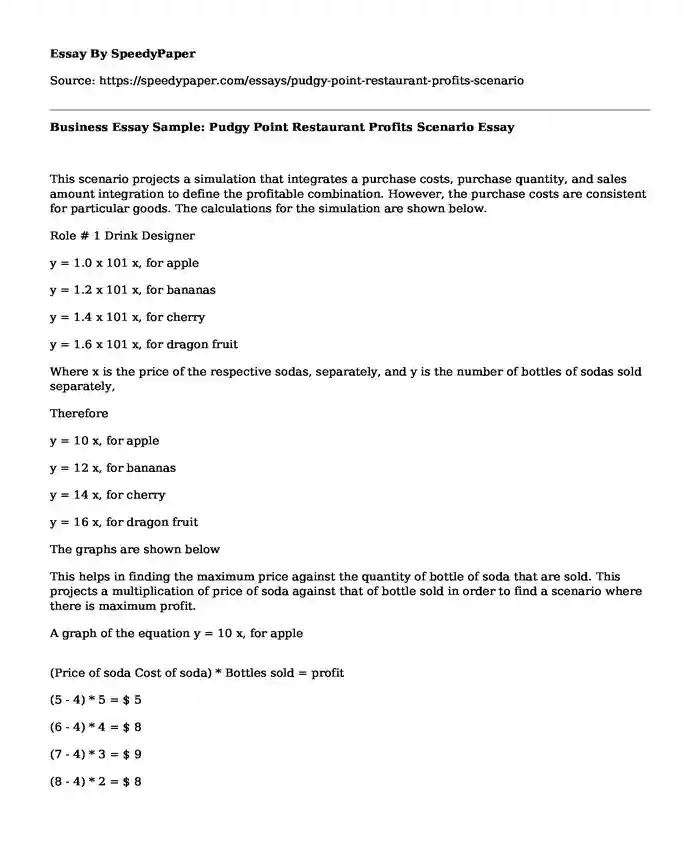# Business Essay Sample: Pudgy Point Restaurant Profits Scenario

Published: 2019-12-09Type of paper: Essay Categories: Finance Business Pages: 2 Wordcount: 455 words
143 views

This scenario projects a simulation that integrates a purchase costs, purchase quantity, and sales amount integration to define the profitable combination. However, the purchase costs are consistent for particular goods. The calculations for the simulation are shown below.Is your time best spent reading someone else’s essay? Get a 100% original essay FROM A CERTIFIED WRITER!

Role # 1 Drink Designer

y = 1.0 x 101 x, for apple

y = 1.2 x 101 x, for bananas

y = 1.4 x 101 x, for cherry

y = 1.6 x 101 x, for dragon fruit

Where x is the price of the respective sodas, separately, and y is the number of bottles of sodas sold separately,

Therefore

y = 10 x, for apple

y = 12 x, for bananas

y = 14 x, for cherry

y = 16 x, for dragon fruit

The graphs are shown below

This helps in finding the maximum price against the quantity of bottle of soda that are sold. This projects a multiplication of price of soda against that of bottle sold in order to find a scenario where there is maximum profit.

A graph of the equation y = 10 x, for apple

(Price of soda Cost of soda) * Bottles sold = profit

(5 - 4) * 5 = \$ 5

(6 - 4) * 4 = \$ 8

(7 - 4) * 3 = \$ 9

(8 - 4) * 2 = \$ 8

(9 - 4) * 1 = \$ 5

(10 - 4) * 0 = \$ 0

Therefore, there will be maximum profit when the price is \$ 7, which is gives a profit of \$ 9

A graph of the equation y = 12 x, for bananas

(Price of soda Cost of soda) * Bottles sold = profit

(4 - 3) * 8 = \$ 8

(5 - 3) * 7 = \$ 14

(6 - 3) * 6 = \$ 18

(7 - 3) * 5 = \$ 20

(8 - 3) * 4 = \$ 20

(9 - 3) * 3 = \$ 18

The Maximum Profit is achieved in two scenarios, where the price of soda is set at \$ 7 and \$ 8, with both \$ 20 profit.

A graph of the equation y = 14 x, for cherry

(Price of soda Cost of soda) * Bottles sold = profit

(3 2) * 11 = \$ 11

(4 2) * 10 = \$ 20

(5 2) * 9 = \$ 27

(6 2) * 8 = \$ 32

(7 2) * 7 = \$ 35

(8 2) * 6 = \$ 36

(9 2) * 5 = \$ 35

(10 2) * 4 = \$ 32

The Maximum profit is achieved when the selling price is set at \$ 8

A graph of the equation y = 16 x, for dragon fruit

(Price of soda Cost of soda) * Bottles sold = profit

(2 - 1) * 14 = \$14

(3 - 1) * 13 = \$26

(4 - 1) * 12 = \$36

(5 - 1) * 11 = \$44

(6 - 1) * 10 = \$50

(7 - 1) * 9 = \$54

(8 - 1) * 8 = \$56

(9 - 1) * 7 = \$56

(10 - 1) * 6 = \$54

The Maximum profit is achievable when the price of soda is set at two scenarios, that is, \$ 8 and \$ 9 and gives a profit of \$ 56.

Scenario questions

Question 1

I have understood how to integrate math, into simulating a business scenario through understanding general aspects of conducting experiments in real life through mathematics.

Question 2

Based on the fact that the simulations prospect a realistic world, they will help me understand the appropriateness of independence through self-employment. I will not have the urge for being employed by an organization but rather manage a business.

Question 3

I will adopt basic error stipulations and other business scenarios like discounts and the possibility of discounts, in aspects of probability and its relation to standard deviation. Through this, I will have a more accurate projection of the scenario, because this is a possibility in business.

Question 4

I have excellent understanding of simulation, however, based on the fact that I am yet to learn more from similar situation and encounter complex scenarios, I will require more exposure to more complex scenarios in order to achieve this prospect.